# 信号计算器

10 五月 2016, 11:46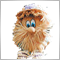0
5 535

### 2. 信号计算器的使用手册

2.1. 开始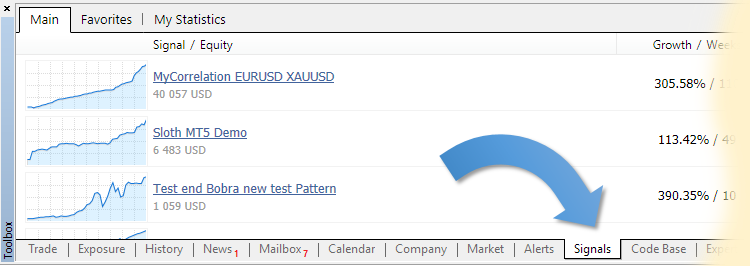"信号"页面应该在连接交易账户之后激活，或者在连接另一个交易账户时激活。

2.2. 用户界面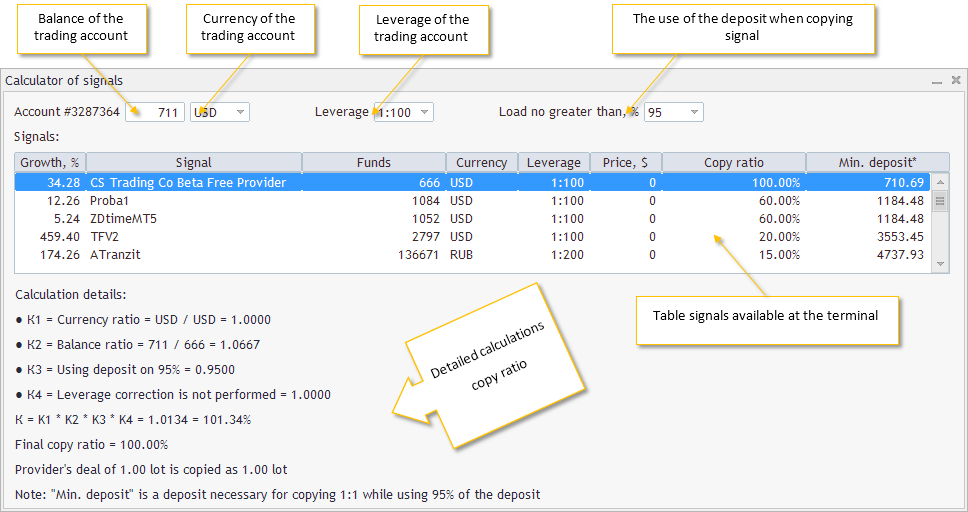• Trading account's balance(交易账户余额) — 当前所连接的交易账户的余额 — 可编辑栏位。在把信号计算器附加到图表之后或者改变了图表的时段，"Balance(余额)"栏位就包含了那个时刻它所连接的交易账户的余额。这个栏位只允许输入0到9的数字。
• Trading account's currency(交易账户币别) — 当前所连接的交易账户的币别 — 一个常用币别的下拉列表。在把信号计算器附加到图表之后或者改变了图表的时段，计算器会尝试在列表中找到交易账户的币别名称。如果没有找到对应的币别，就会选择"USD"作为默认值。
• Trading account's leverage(交易账户杠杆) — 当前所连接的交易账户的杠杆 - 一个常用杠杆的下拉列表。在把信号计算器附加到图表之后或者改变了图表的时段，计算器会尝试在列表中寻找当前连接账户的杠杆。如果没有找到对应值, 就使用"1:100"的杠杆作为默认值。
• Deposit load when copying a signal(复制信号时的入金比例) — 一个复制信号使用入金比例的下拉列表，对应了终端菜单中的"工具" - "选项" - "信号"中的数值，在把信号计算器附加到图表或者改变了图表的时段时，计算器会寻找交易账户的使用入金比例。如果没有找到对应值，就会使用"95%"作为默认使用入金的比例。
• Table of signals available in the terminal(终端中可用的信号表格) — 一个包含"信号"页面所包含信号的表格。表格中的内容根据"Copy ratio(复制比例)"列进行降序排列。表格中的列:
• "Growth, %(增长百分率)" 列 — 通过交易操作账户增长的百分比。
• "Signal(信号)" 列 — 交易信号的名称。
• "Funds(资金)" 列 — 信号提供者账户的自有资金数量，可以考虑其可信度。
• "Currency(币别)" 列 — 信号提供者交易账户的币别。
• "Leverage(杠杆)" 列 — 信号提供者交易账户的杠杆。
• "Price, \$(价格)" 列 — 交易信号的价格，单位为美元。
• The "最小存款 *" 列 — 当使用95%资金时，达到1:1复制所需的存款数量。说得更清楚一些，这就是在选择使用"95%"的存款的条件下，把信号提供者的交易按照1:1复制到您的交易账户所需要的存款数量。
• Detailed calculation of copy ratio (复制比例的计算细节) — 计算所选信号复制比例的每一步骤的计算过程。
• К1 — 您的交易账户与信号提供者交易账户币别的比例。
• К2 — 您的交易账户与信号提供者交易账户余额的比例。
• К3 — 使用存款的比例，把百分数转换为比例。
• К4 — 杠杆差异的修正比例。
• К — 最终比例，它是用比例相乘 К1*К2*К3*К4 计算所得。
• 最终复制比例 — 使用多步算法对К比例进行取整

2.3. 获取复制比例

2.4. 复制比例的计算细节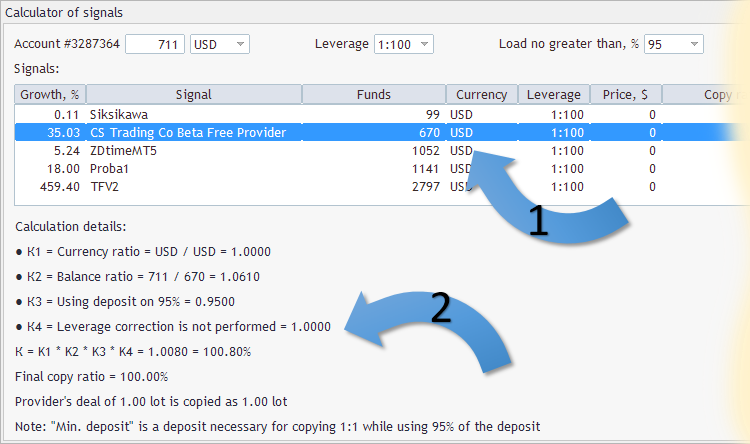2.5. 无法映射

```Сalculator for signals (EURUSD,M5)      Error find symbols: (Account currency SGD, Signal currency RUB)
Сalculator for signals (EURUSD,M5)      Error find symbols: (Account currency SGD, Signal currency EUR)
Сalculator for signals (EURUSD,M5)      Error find symbols: (Account currency SGD, Signal currency EUR)

```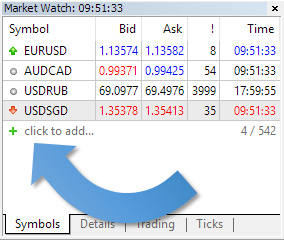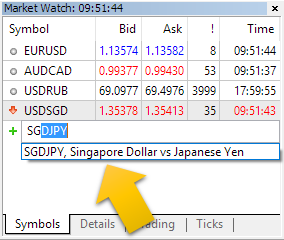### 3. 开发信号计算器

3.1. 界面设计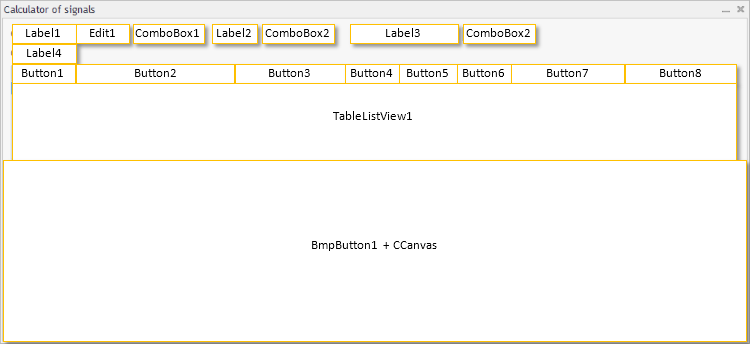```//+------------------------------------------------------------------+
//| 定义                               |
//+------------------------------------------------------------------+
//--- 缩进和间隙
#define INDENT_LEFT                         (11)      // 左边的缩进 (包含边框宽度)
#define INDENT_TOP                          (11)      // 顶端的缩进 (包含边框宽度)
#define CONTROLS_GAP_Y                      (5)       // 纵坐标上的间隙
//--- for combo boxes
#define COMBOBOX_WIDTH                      (60)      // 横坐标上的大小
#define COMBOBOX_HEIGHT                     (20)      // 纵坐标上的大小
//--- for list view
#define LIST_HEIGHT                         (102)     // 纵坐标上的大小
//--- for buttons
#define BUTTON_WIDTH                        (72)      // 横坐标上的大小
#define BUTTON_HEIGHT                       (20)      // 纵坐标上的大小
//--- for the indication area
#define EDIT_WIDTH                          (60)      // 横坐标上的大小
#define EDIT_HEIGHT                         (20)      // 纵坐标上的大小

```

1. 第一行 — Label1, Edit1, ComboBox1, Label2, ComboBox2, Label3, ComboBox2
2. 第二行 — Label 4
3. 第三行 — 按钮 Button1- Button8
4. 第四行 — 新控件 — TableListView1
5. 第五行 — BmpButton1 对象，它是基于CCanvans以显示位图的方式来显示控件的。

```//+------------------------------------------------------------------+
//| 创建                                |
//+------------------------------------------------------------------+
bool CoSDialog::Create(const long chart,const string name,const int subwin,const int x1,const int y1,const int x2,const int y2)
{
//---
m_error=true;
if(!CAppDialog::Create(chart,name,subwin,x1,y1,x2,y2))
return(false);
//---

```

```//+------------------------------------------------------------------+
//| 创建 "Signals" 标签                         |
//+------------------------------------------------------------------+
bool CoSDialog::CreateLabel4(void)
{
//--- 坐标
int x1=INDENT_LEFT;
int y1=INDENT_TOP+COMBOBOX_HEIGHT+CONTROLS_GAP_Y;
int x2=x1+100;
int y2=y1+COMBOBOX_HEIGHT;
//--- 创建
if(!m_label4.Create(m_chart_id,m_name+"Label4",m_subwin,x1,y1,x2,y2))
return(false);
if(!m_label4.Text(m_languages.GetText(3)))
return(false);
return(false);
//--- 成功
return(true);
}

```

```   if(!m_label4.Create(m_chart_id,m_name+"Label4",m_subwin,x1,y1,x2,y2))
return(false);

```

```   if(!Add(m_label4))
return(false);

```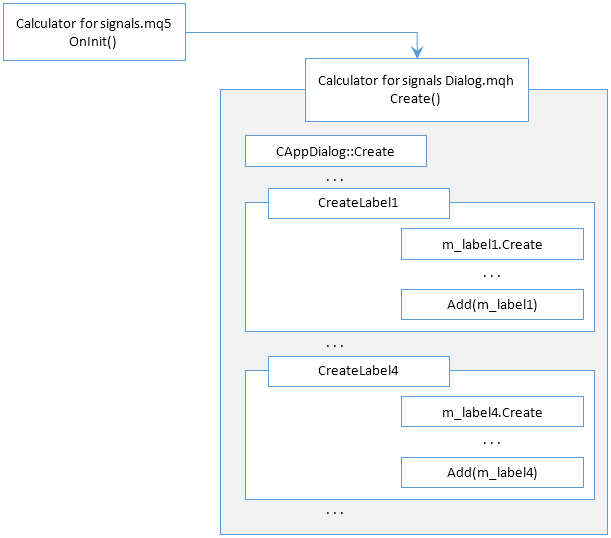• 创建面板 (CAppDialog::Create)
• 按照以下顺序创建控件:
• 创建元件 (element_N.Create)
• 修改元件属性

3.2. 在面板上创建画布

```//--- 创建画布
if(!m_canvas1.Create("Canvas1",x2,y2,COLOR_FORMAT_XRGB_NOALPHA))
{
Print("Error creating canvas: ",GetLastError());
return(false);
}
m_canvas1.FontSet("Trebuchet MS",-100,FW_THIN);
m_canvas1.Erase(ColorToARGB(C'0xF7,0xF7,0xF7',255));
m_canvas1.Update(true);

```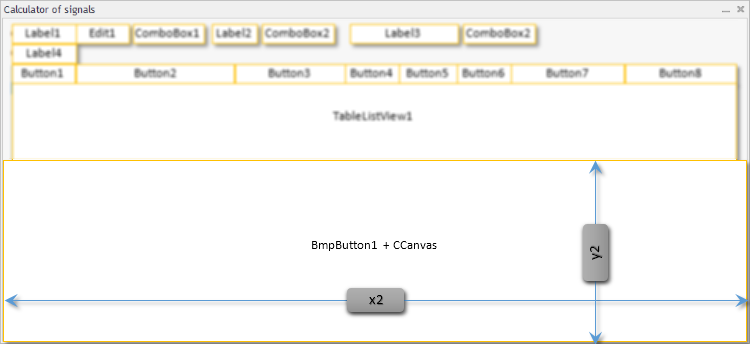```//--- 创建
if(!m_bmp_button1.Create(m_chart_id,m_name+"BmpButton1",m_subwin,x1,y1,x1+10,y1+10))
return(false);

```

m_bmp_button1控件创建时的大小是10*10像素点，尺寸较小也没有关系，真正的坐标x1y1是关键的，它们是锚点。当控件大小适应图标大小时，这些坐标(x1; y1)是起作用的。

```//--- 设置 CBmpButton 控件位图文件的名称
if(!m_bmp_button1.BmpOnName(m_canvas1.ResourceName()))
return(false);

```

```   if(!Add(m_bmp_button1))
return(false);

```

3.3. 二维数组排序

Name Copy ratio
TestUSD 15.0
Zeus PRO 15.0
CS Trading Co Beta Free Provider 1510.0
Mint blueberry ice cream 8.0
MT5Hedging 7.0
Hedge 1.0
Siksikawa 8770.0
Week H4 15.0
WGT Live Signal 45.0
MOsg style 6.0

name rate  min_deposit
Insert : MyCorrelation EURUSD XAUUSD  7  133134.7143
row #0: MyCorrelation EURUSD XAUUSD  7  133134.7143
Insert : EA microPatience AvzPrecio v6 HG 10  7  133134.7143
row #0: MyCorrelation EURUSD XAUUSD  7  133134.7143
row #1: EA microPatience AvzPrecio v6 HG 10  7  133134.7143
Insert : EURUSD Daytrade  170  5482.017647
row #0: EURUSD Daytrade  170  5482.017647
row #1: MyCorrelation EURUSD XAUUSD  7   133134.7143
row #2: EA microPatience AvzPrecio v6 HG 10  7  133134.7143
Insert : Exp TickSniper PRO FULL MT5  50  18638.86
row #0: EURUSD Daytrade  170  5482.017647
row #1: Exp TickSniper PRO FULL MT5  50  18638.86
row #2: MyCorrelation EURUSD XAUUSD  7  133134.7143
row #3: EA microPatience AvzPrecio v6 HG 10  7  133134.7143
Insert : Example1  3  310647.6667
row #0: EURUSD Daytrade  170  5482.017647
row #1: Exp TickSniper PRO FULL MT5  50  18638.86
row #2: MyCorrelation EURUSD XAUUSD  7  133134.7143
row #3: EA microPatience AvzPrecio v6 HG 10  7  133134.7143
row #4: Example1  3  310647.6667
Insert : Big sale  80  11649.2875
row #0: EURUSD Daytrade  170  5482.017647
row #1: Big sale  80  11649.2875
row #2: Exp TickSniper PRO FULL MT5  50  18638.86
row #3: MyCorrelation EURUSD XAUUSD  7  133134.7143
row #4: EA microPatience AvzPrecio v6 HG 10  7  133134.7143
row #5: Example1  3  310647.6667

```         else
{
min_deposit=AccInfo.balance/rate*100.0;
}
//Print("Insert : ",name,"; ",rate,"; ",min_deposit);
m_table.InsertSort(new CLineTable(name,rate,min_deposit));
//for(int m=0;m<m_table.Total();m++)
//  {
//   CLineTable *line=m_table.At(m);
//   Print("row #",m,": ",line.Text(),"; ",line.Number(),"; ",line.Number1());
//  }
}
else PrintFormat("Error in call of SignalBaseSelect. Error code=%d",GetLastError());
}
//---
return(true);
}

```

3.4. 新控件 — CTableListView 表格

CTableListView控件表格包含两个部分 — 一个用户可以看到和操作的可见部分，以及保存表格单元数据的不可见部分。

```   virtual bool      Create(const long chart,const string name,const int subwin,const int x1,
const int y1,const int x2,const int y2,const uchar columns,const ushort &columns_size[]);

```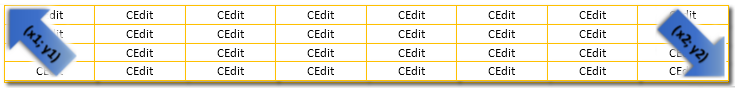`   CArrayObj         m_arr_rows;             // objects-rows (CEdit) 指针数组`

(一篇关于指针使用的文章 — 在 MQL5 中使用对象指针)

m_arr_rows指针数组就像一个两层的俄罗斯娃娃那样工作，第一层把指针保存在row对象中，它保存了表格单元对象(CEdit元件)的指针: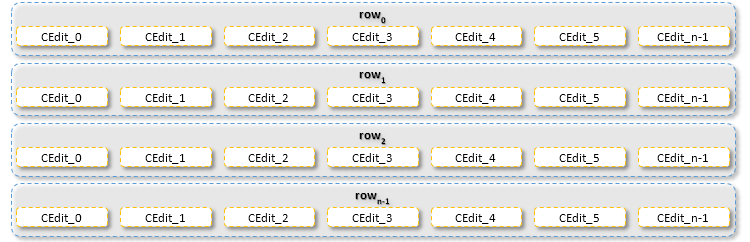```   CArrayObj         m_arr_rows_str;         // objects-rows (CArrayString) 的指针数组
CArrayObj         m_arr_rows_val;         // objects-rows (CArrayLong) 的指针数组

```

m_arr_rows_str 指针数组和m_arr_rows_val 的结构与m_arr_rows指针数组的结构类似, 所不同的就是分别使用了CArrayStringCArrayLong

3.4.1. 操作动态指针数组的例子

```//+------------------------------------------------------------------+
//| 创建控件                               |
//+------------------------------------------------------------------+
bool CTableListView::Create(const long chart,const string name,const int subwin,const int x1,
const int y1,const int x2,const int y2,const uchar columns,const ushort &columns_size[])
{
m_columns=columns;
ArrayResize(m_columns_size,m_columns);
if(ArraySize(columns_size)!=m_columns)
return(false);
ArrayCopy(m_columns_size,columns_size,0,0,WHOLE_ARRAY);
m_columns_size-=1;
m_columns_size[m_columns-1]-=1;
int y=y2;
//--- 如果之前确定了可见的行数，调整垂直大小
if(!TotalView((y2-y1)/m_item_height))
y=m_item_height+y1+2*CONTROLS_BORDER_WIDTH;
//--- 检查可见行数
if(m_total_view<1)
return(false);
//--- 调用父类方法
if(!CWndClient::Create(chart,name,subwin,x1,y1,x2,y))
return(false);
//--- 设置
if(!m_background.ColorBackground(CONTROLS_LIST_COLOR_BG))
return(false);
if(!m_background.ColorBorder(CONTROLS_LIST_COLOR_BORDER))
return(false);
//--- 创建依赖的控件
CArrayObj *m_arr_cells;
for(int i=0;i<m_total_view;i++)
{
m_arr_cells=new CArrayObj;
if(CheckPointer(m_arr_cells)==POINTER_INVALID)
return(false);
for(int j=0;j<m_columns;j++)
{
CEdit *m_cell;
m_cell=new CEdit;
if(CheckPointer(m_cell)==POINTER_INVALID)
return(false);
}
}
//---
for(int i=0;i<m_total_view;i++)
{
if(!CreateRow(i))
return(false);
if(m_height_variable && i>0)
{
// m_rows[i].Hide(); ///
CArrayObj *m_arr_cells_i=m_arr_rows.At(i);
if(CheckPointer(m_arr_cells_i)==POINTER_INVALID)
return(false);
for(int j=0;j<m_arr_cells_i.Total();j++)
{
CEdit *m_cell=m_arr_cells_i.At(j);
if(CheckPointer(m_cell)==POINTER_INVALID)
return(false);
if(!m_cell.Hide())
return(false);
}
}
}
//--- 成功
return(true);
}

```

```//--- 如果之前确定了可见的行数，调整垂直大小
if(!TotalView((y2-y1)/m_item_height))
y=m_item_height+y1+2*CONTROLS_BORDER_WIDTH;

```

```//--- 创建依赖的控件
CArrayObj *m_arr_cells;
for(int i=0;i<m_total_view;i++)
{
m_arr_cells=new CArrayObj;
if(CheckPointer(m_arr_cells)==POINTER_INVALID)
return(false);
for(int j=0;j<m_columns;j++)
{
CEdit *m_cell;
m_cell=new CEdit;
if(CheckPointer(m_cell)==POINTER_INVALID)
return(false);
}
}
//---

```

`      m_arr_rows.Add(m_arr_cells);`

```//---
for(int i=0;i<m_total_view;i++)
{
if(!CreateRow(i))
return(false);
.
.
.
}
//--- 成功
return(true);

```

CreateRow 方法:

```//+------------------------------------------------------------------+
//| 创建 "row(行)"                            |
//+------------------------------------------------------------------+
bool CTableListView::CreateRow(const int index)
{
.
.
.
//--- 创建
CArrayObj *m_arr_cells=m_arr_rows.At(index);
if(CheckPointer(m_arr_cells)==POINTER_INVALID)
return(false);
for(int i=0;i<m_arr_cells.Total();i++)
{
CEdit *m_cell=m_arr_cells.At(i);
if(CheckPointer(m_cell)==POINTER_INVALID)
return(false);
x1+=x2;
x2=m_columns_size[i];
if(!m_cell.Create(m_chart_id,m_name+"_"+IntegerToString(index)+"_"+IntegerToString(i),
m_subwin,x1,y1,x1+x2,y2))
return(false);
if(!m_cell.Text(""))
return(false);
return(false);
return(false);
}
.
.
.
return(true);
}

```

```   .
//--- 创建
CArrayObj *m_arr_cells=m_arr_rows.At(index);
if(CheckPointer(m_arr_cells)==POINTER_INVALID)
return(false);
for(int i=0;i<m_arr_cells.Total();i++)

```

```      CEdit *m_cell=m_arr_cells.At(i);
if(CheckPointer(m_cell)==POINTER_INVALID)
return(false);

```

```      if(!m_cell.Create(m_chart_id,m_name+"_"+IntegerToString(index)+"_"+IntegerToString(i),
m_subwin,x1,y1,x1+x2,y2))
return(false);

```

```      if(!m_cell.Text(""))
return(false);
return(false);

```

```      if(!Add(m_cell))
return(false);
}

```

### 结论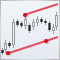MQL5酷客宝典 - 滑动通道编程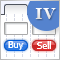图形界面 IV: 多窗口模式与优先级系统 (第二章)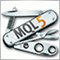通用EA:自定义策略和辅助交易类(第三章)通用智能交易系统:组合交易及管理策略组合(第四章)